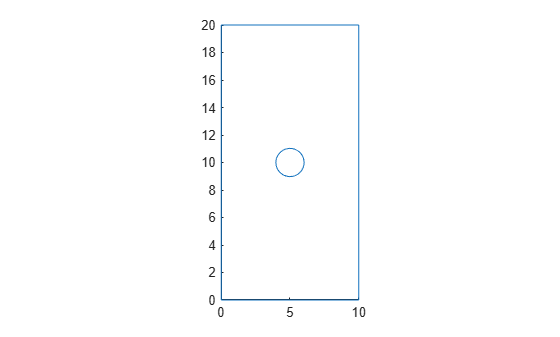# findElements

Find mesh elements in specified region

## Syntax

``elemIDs = findElements(mesh,"region",RegionType,RegionID)``
``elemIDs = findElements(mesh,"box",xlim,ylim)``
``elemIDs = findElements(mesh,"box",xlim,ylim,zlim)``
``elemIDs = findElements(mesh,"radius",center,radius)``
``elemIDs = findElements(mesh,"attached",nodeID)``

## Description

example

````elemIDs = findElements(mesh,"region",RegionType,RegionID)` returns the IDs of the mesh elements that belong to the specified geometric region.```
````elemIDs = findElements(mesh,"box",xlim,ylim)` returns the IDs of the mesh elements within a bounding box specified by `xlim` and `ylim`. Use this syntax for 2-D meshes.```

example

````elemIDs = findElements(mesh,"box",xlim,ylim,zlim)` returns the IDs of the mesh elements located within a bounding box specified by `xlim`, `ylim`, and `zlim`. Use this syntax for 3-D meshes.```

example

````elemIDs = findElements(mesh,"radius",center,radius)` returns the IDs of mesh elements located within a circle (for 2-D meshes) or sphere (for 3-D meshes) specified by `center` and `radius`.```

example

````elemIDs = findElements(mesh,"attached",nodeID)` returns the IDs of the mesh elements attached to the specified node. Here, `nodeID` is the ID of a corner node. This syntax ignores the IDs of the nodes located in the middle of element edges.For multiple nodes, specify `nodeID` as a vector.```

## Examples

collapse all

Find the elements associated with a geometric region.

Create a PDE model.

`model = createpde;`

Include the geometry of the built-in function `lshapeg`. Plot the geometry.

```geometryFromEdges(model,@lshapeg); pdegplot(model,FaceLabels="on",EdgeLabels="on")```Generate a mesh.

`mesh = generateMesh(model,Hmax=0.5);`

Find the elements associated with face 2.

`Ef2 = findElements(mesh,"region",Face=2);`

Highlight these elements in green on the mesh plot.

```figure pdemesh(mesh,ElementLabels="on") hold on pdemesh(mesh.Nodes,mesh.Elements(:,Ef2),EdgeColor="green")```Find the elements located within a specified box.

Create a PDE model.

`model = createpde;`

Import and plot the geometry.

```importGeometry(model,"PlateHolePlanar.stl"); pdegplot(model)```Generate a mesh.

`mesh = generateMesh(model,"Hmax",2,"Hmin",0.4)`
```mesh = FEMesh with properties: Nodes: [2x386 double] Elements: [6x172 double] MaxElementSize: 2 MinElementSize: 0.4000 MeshGradation: 1.5000 GeometricOrder: 'quadratic' ```

Find the elements located within the following box.

`Eb = findElements(mesh,"box",[5 10],[10 20]);`

Highlight these elements in green on the mesh plot.

```figure pdemesh(model) hold on pdemesh(mesh.Nodes,mesh.Elements(:,Eb),"EdgeColor","green")```Find the elements located within a specified disk.

Create a PDE model.

`model = createpde;`

Import and plot the geometry.

```importGeometry(model,"PlateHolePlanar.stl"); pdegplot(model)```Generate a mesh.

`mesh = generateMesh(model,"Hmax",2,"Hmin",0.4,"GeometricOrder","linear")`
```mesh = FEMesh with properties: Nodes: [2x107 double] Elements: [3x172 double] MaxElementSize: 2 MinElementSize: 0.4000 MeshGradation: 1.5000 GeometricOrder: 'linear' ```

Find the elements located within radius 2 from the center [5,10].

`Er = findElements(mesh,"radius",[5 10],2);`

Highlight these elements in green on the mesh plot.

```figure pdemesh(model) hold on pdemesh(mesh.Nodes,mesh.Elements(:,Er),"EdgeColor","green")```Find the elements attached to a specified corner node.

Create a PDE model.

`model = createpde;`

Import and plot the geometry.

```importGeometry(model,"PlateHolePlanar.stl"); pdegplot(model)```Generate a linear triangular mesh by setting the geometric order value to `linear`. This mesh contains only corner nodes.

```mesh = generateMesh(model,"Hmax",2,"Hmin",0.4, ... "GeometricOrder","linear");```

Find the node closest to the point [15;10].

`N_ID = findNodes(mesh,"nearest",[15;10])`
```N_ID = 10 ```

Find the elements attached to this node.

`En = findElements(mesh,"attached",N_ID)`
```En = 1×3 95 97 98 ```

Highlight the node and the elements in green on the mesh plot.

```figure pdemesh(model) hold on plot(mesh.Nodes(1,N_ID),mesh.Nodes(2,N_ID),"or","Color","g", ... "MarkerFaceColor","g") pdemesh(mesh.Nodes,mesh.Elements(:,En),"EdgeColor","green")```## Input Arguments

collapse all

Mesh object, specified as the `Mesh` property of a `PDEModel` object or as the output of `generateMesh`.

Example: `model.Mesh`

Geometric region type, specified as `"Cell"` or `"Face"`.

Example: `findElements(mesh,"region","Face",1:3)`

Data Types: `char`

Geometric region ID, specified as a vector of positive integers. Find the region IDs by using `pdegplot`.

Example: `findElements(mesh,"region","Face",1:3)`

Data Types: `double`

x-limits of the bounding box, specified as a two-element row vector. The first element of `xlim` is the lower x-bound, and the second element is the upper x-bound.

Example: ```findElements(mesh,"box",[5 10],[10 20])```

Data Types: `double`

y-limits of the bounding box, specified as a two-element row vector. The first element of `ylim` is the lower y-bound, and the second element is the upper y-bound.

Example: ```findElements(mesh,"box",[5 10],[10 20])```

Data Types: `double`

z-limits of the bounding box, specified as a two-element row vector. The first element of `zlim` is the lower z-bound, and the second element is the upper z-bound. You can specify `zlim` only for 3-D meshes.

Example: ```findElements(mesh,"box",[5 10],[10 20],[1 2])```

Data Types: `double`

Center of the bounding circle or sphere, specified as a two-element row vector for a 2-D mesh or three-element row vector for a 3-D mesh. The elements of these vectors contain the coordinates of the center of a circle or a sphere.

Example: ```findElements(mesh,"radius",[0 0 0],0.5)```

Data Types: `double`

Radius of the bounding circle or sphere, specified as a positive number.

Example: ```findElements(mesh,"radius",[0 0 0],1)```

Data Types: `double`

ID of the corner node of the element, specified as a positive integer or a vector of positive integers. The `findElements` function can find an ID of the element by the ID of the corner node of the element. The function ignores IDs of the nodes located in the middle of element edges. For multiple nodes, specify `nodeID` as a vector.

Example: ```findElements(mesh,"attached",[7 8 21])```

Data Types: `double`

## Output Arguments

collapse all

Element IDs, returned as a positive integer or a row vector of positive integers.

## Version History

Introduced in R2018a